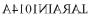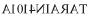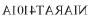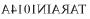Courses

# Directions: In each of the following questions, there is combination of alphabet or number followed by four alternatives. Choose the alternative which most clearly resembles the mirror image of the given combination.TARAIN1014Aa)b)c)d)Correct answer is option 'D'. Can you explain this answer? Related Test: Olympiad Test: Mirror Images -1

## Class 6 QuestionAyush Kumar 4 weeks ago
D is correct

This discussion on Directions: In each of the following questions, there is combination of alphabet or number followed by four alternatives. Choose the alternative which most clearly resembles the mirror image of the given combination.TARAIN1014Aa)b)c)d)Correct answer is option 'D'. Can you explain this answer? is done on EduRev Study Group by Class 6 Students. The Questions and Answers of Directions: In each of the following questions, there is combination of alphabet or number followed by four alternatives. Choose the alternative which most clearly resembles the mirror image of the given combination.TARAIN1014Aa)b)c)d)Correct answer is option 'D'. Can you explain this answer? are solved by group of students and teacher of Class 6, which is also the largest student community of Class 6. If the answer is not available please wait for a while and a community member will probably answer this soon. You can study other questions, MCQs, videos and tests for Class 6 on EduRev and even discuss your questions like Directions: In each of the following questions, there is combination of alphabet or number followed by four alternatives. Choose the alternative which most clearly resembles the mirror image of the given combination.TARAIN1014Aa)b)c)d)Correct answer is option 'D'. Can you explain this answer? over here on EduRev! Apart from being the largest Class 6 community, EduRev has the largest solved Question bank for Class 6.

### Related Content

This discussion on Directions: In each of the following questions, there is combination of alphabet or number followed by four alternatives. Choose the alternative which most clearly resembles the mirror image of the given combination.TARAIN1014Aa)b)c)d)Correct answer is option 'D'. Can you explain this answer? is done on EduRev Study Group by Class 6 Students. The Questions and Answers of Directions: In each of the following questions, there is combination of alphabet or number followed by four alternatives. Choose the alternative which most clearly resembles the mirror image of the given combination.TARAIN1014Aa)b)c)d)Correct answer is option 'D'. Can you explain this answer? are solved by group of students and teacher of Class 6, which is also the largest student community of Class 6. If the answer is not available please wait for a while and a community member will probably answer this soon. You can study other questions, MCQs, videos and tests for Class 6 on EduRev and even discuss your questions like Directions: In each of the following questions, there is combination of alphabet or number followed by four alternatives. Choose the alternative which most clearly resembles the mirror image of the given combination.TARAIN1014Aa)b)c)d)Correct answer is option 'D'. Can you explain this answer? over here on EduRev! Apart from being the largest Class 6 community, EduRev has the largest solved Question bank for Class 6.